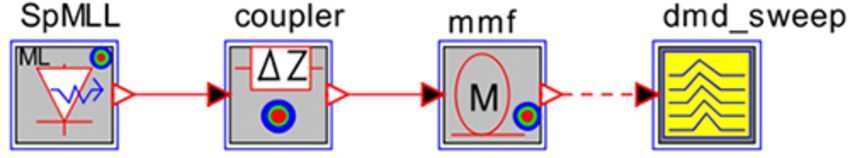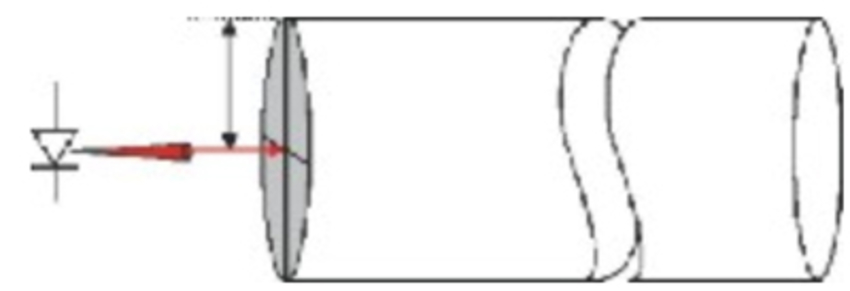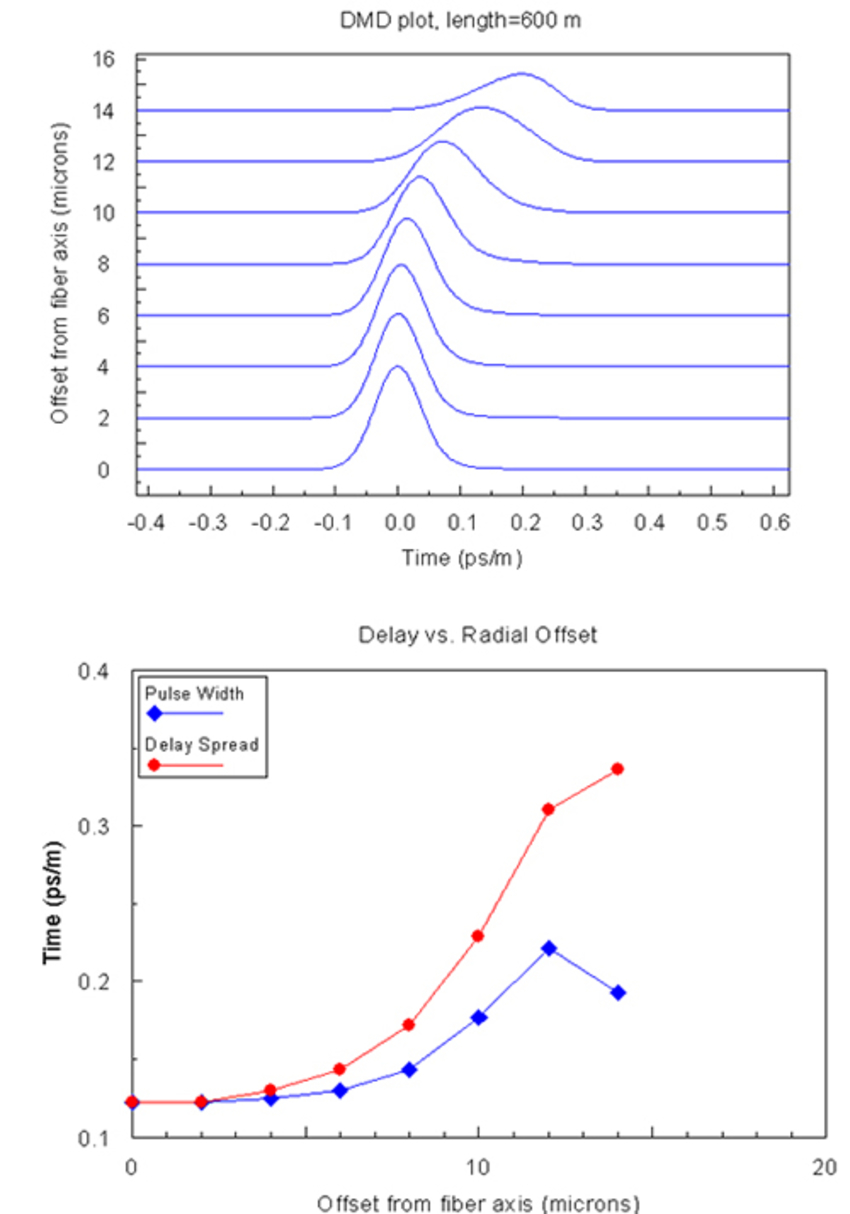# Differential Mode Delay (DMD)

## Tool Used: ModeSYS

This example demonstrates using ModeSYS for DMD measurement. Figure below shows a simple topology used to measure the DMD of a multimode fiber:Since DMD is a measure of the fiber's spatio-temporal impulse response, it is important to use an input pulse that approximates a delta function in both space and time. The DMD measurement is performed by scanning the optical source across the face of the fiber as shown below:

Basically, the DMD is simulated by using the spatial coupler to shift the laser input by a distance specified by the variable that is to be scanned at specified interval. The DMD analyzer tool has been designed to store the temporal simulation results after each parameter scanning run, then to plot the aggregate results after the last scan as shown below:The DMD analyzer also plots DMD vs Radial offset plot as shown below: